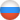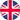Читать статью 'Goertzel algorithm for signals spectral analysis' в журнале Software systems and computational methods на сайте nbpublish.comРусEngDuring last 365 days Approved articles: 1717,   Articles in work: 352 Declined articles: 409
•••• Authors
••• Reviewing
••Library

## Galanina N.A., Dmitriev D.D., Akhmetzyanov D.I. Goertzel algorithm for signals spectral analysis

Published in journal "Software systems and computational methods", 2013-4 in rubric "Mathematical models and computer simulation experiment", pages 376-383.

Resume: the article presents results of software realization of Goertzel algorithm for determining the phase shift between two sinusoidal signals. The authors show practical appliance of Goertzel algorithm for calculation of active component R of the complex impedance filter Z. It is noted that the Goertzel algorithm is implemented in form of the filter with infinite impulse response (IIR-filter) of the second order with two real feedback coefficients and one complex coefficient in the forward loop. Modeling was carried out in the MathCad computer algebra system. The article stated that the Goertzel algorithm allows to efficiently calculate fixed spectral counts of the discrete Fourier transform without the calculation of the Fourier transform itself. The authors point out that the Goertzel algorithm proved to be eff ective for computation of the spectral components at high sampling rates and correct calculation of sampling counts. In the Mathcad the authors built a model showing the working capacity of this algorithm. Using this model the optimal parameters for further practical implementation of the Goertzel algorithm were found.

Keywords: Goertzel algoritm, phase shift, sinusoidal signals, complex impedance, feedback, direct link chain, Mathcad, modeling, sampling, fixed spectral counts

DOI: 10.7256/2305-6061.2013.4.10543